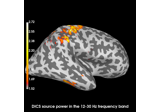# mne.compute_rank#

mne.compute_rank(inst, rank=None, scalings=None, info=None, tol='auto', proj=True, tol_kind='absolute', on_rank_mismatch='ignore', verbose=None)[source]#

Compute the rank of data or noise covariance.

This function will normalize the rows of the data (typically channels or vertices) such that non-zero singular values should be close to one.

Parameters
instinstance of `Raw`, `Epochs`, or `Covariance`

Raw measurements to compute the rank from or the covariance.

rank`None` | ‘info’ | ‘full’ | `dict`

This controls the rank computation that can be read from the measurement info or estimated from the data. When a noise covariance is used for whitening, this should reflect the rank of that covariance, otherwise amplification of noise components can occur in whitening (e.g., often during source localization).

`None`

The rank will be estimated from the data after proper scaling of different channel types.

`'info'`

The rank is inferred from `info`. If data have been processed with Maxwell filtering, the Maxwell filtering header is used. Otherwise, the channel counts themselves are used. In both cases, the number of projectors is subtracted from the (effective) number of channels in the data. For example, if Maxwell filtering reduces the rank to 68, with two projectors the returned value will be 66.

`'full'`

The rank is assumed to be full, i.e. equal to the number of good channels. If a `Covariance` is passed, this can make sense if it has been (possibly improperly) regularized without taking into account the true data rank.

`dict`

Calculate the rank only for a subset of channel types, and explicitly specify the rank for the remaining channel types. This can be extremely useful if you already know the rank of (part of) your data, for instance in case you have calculated it earlier.

This parameter must be a dictionary whose keys correspond to channel types in the data (e.g. `'meg'`, `'mag'`, `'grad'`, `'eeg'`), and whose values are integers representing the respective ranks. For example, `{'mag': 90, 'eeg': 45}` will assume a rank of `90` and `45` for magnetometer data and EEG data, respectively.

The ranks for all channel types present in the data, but not specified in the dictionary will be estimated empirically. That is, if you passed a dataset containing magnetometer, gradiometer, and EEG data together with the dictionary from the previous example, only the gradiometer rank would be determined, while the specified magnetometer and EEG ranks would be taken for granted.

The default is `None`.

scalings

Defaults to `dict(mag=1e15, grad=1e13, eeg=1e6)`. These defaults will scale different channel types to comparable values.

info

The `mne.Info` object with information about the sensors and methods of measurement. Only necessary if `inst` is a `mne.Covariance` object (since this does not provide `inst.info`).

tol`float` | ‘auto’

Tolerance for singular values to consider non-zero in calculating the rank. The singular values are calculated in this method such that independent data are expected to have singular value around one. Can be ‘auto’ to use the same thresholding as `scipy.linalg.orth()`.

projbool

If True, all projs in `inst` and `info` will be applied or considered when `rank=None` or `rank='info'`.

tol_kind`str`

Can be: “absolute” (default) or “relative”. Only used if `tol` is a float, because when `tol` is a string the mode is implicitly relative. After applying the chosen scale factors / normalization to the data, the singular values are computed, and the rank is then taken as:

• `'absolute'`

The number of singular values `s` greater than `tol`. This mode can fail if your data do not adhere to typical data scalings.

• `'relative'`

The number of singular values `s` greater than `tol * s.max()`. This mode can fail if you have one or more large components in the data (e.g., artifacts).

New in version 0.21.0.

on_rank_mismatch`str`

If an explicit MEG value is passed, what to do when it does not match an empirically computed rank (only used for covariances). Can be ‘raise’ to raise an error, ‘warn’ (default) to emit a warning, or ‘ignore’ to ignore.

New in version 0.23.

verbose

Control verbosity of the logging output. If `None`, use the default verbosity level. See the logging documentation and `mne.verbose()` for details. Should only be passed as a keyword argument.

Returns
rank`dict`

Estimated rank of the data for each channel type. To get the total rank, you can use `sum(rank.values())`.

Notes

New in version 0.18.

## Examples using `mne.compute_rank`#Compute evoked ERS source power using DICS, LCMV beamformer, and dSPM

Compute evoked ERS source power using DICS, LCMV beamformer, and dSPM